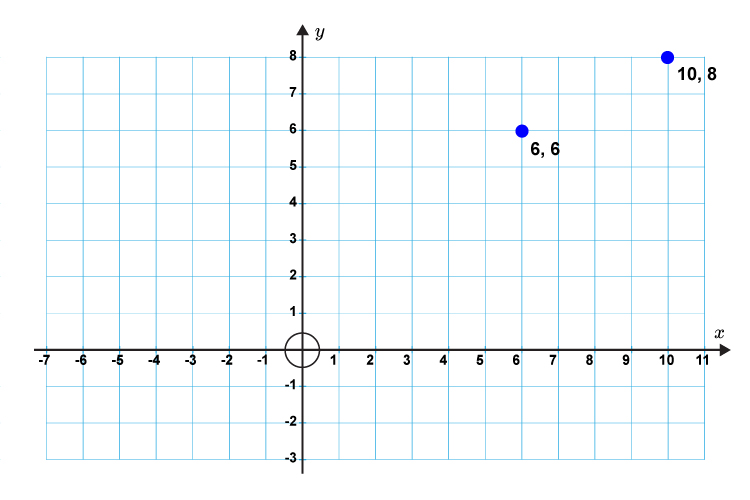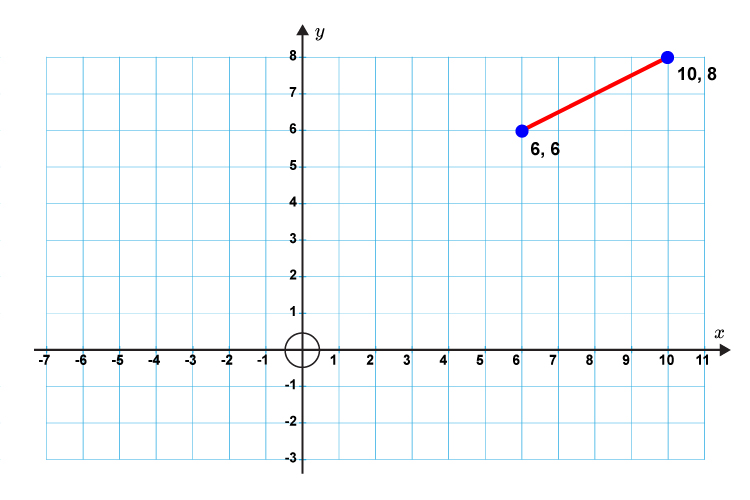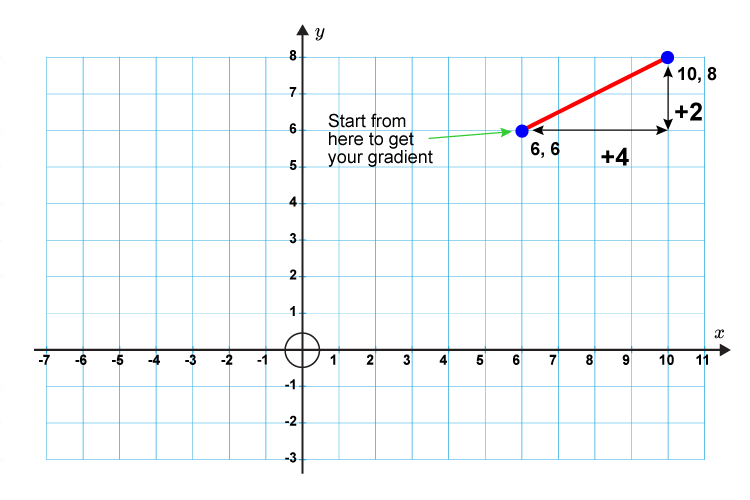# When you’re given coordinates

When you are given coordinates for two points on a straight line finding the gradient is logic based on what you already know.

Example

Given 10, 8 and 6, 6 find the gradient of the line.

First plot on a graph to help visualise.Gradient\ (m)=\(y(chang\e\ i\n))/(x(chang\e\ i\n)

Then draw the line between the points.Now work out the horizontal (x) and vertical (y)Gradient\ (m)=(+2)/(+4)=1/2

Gradient of this line (m)=1/2=0.5